Study P4 Mathematics Maths - Division - Geniebook# Division

In Mathematics, there are four basic arithmetic operations, i.e. addition, subtraction, multiplication and division.

Division means to distribute a value/number/amount equally. Its result may or may not be a whole number. It can also be in the form of a decimal.

1. Division up to 4-digit by 1-digit numbers

## Long Division

Division can be done by the long division method.

Example:

Do the following division.

$$965 \div 8 =$$ __________

1. What is the quotient?

Solution: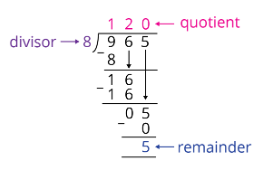120

1. What is the remainder?

5

## 1. Division Of 4-Digit Number By 1-Digit Number

Question 1:

Do the following division.

$$4824 \div 2 =$$ __________

Solution: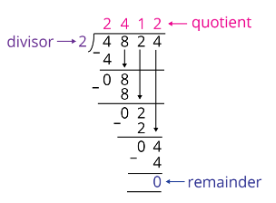2412

Question 2:

Perform the following division.

$$6009 \div 3 =$$ __________

Solution: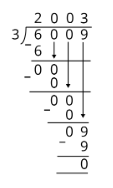2003

Question 3:

Perform the following division.

$$8012 \div 4 =$$ __________

Solution:2003

Question 4:

Perform the following division.

$$5525 \div 5 =$$ _________

Solution:1105

Question 5:

Perform the following division.

$$5649 \div 7 =$$ __________

Solution:807

Question 6:

Perform the following division.

$$2989 \div 4 =$$ __________ $$\text{R}$$ __________

Solution:$$2989 \div 4 = 747 \text{ R } 1$$

747  R  1

Question 7:

Perform the following division.

$$7268 \div 8 =$$ __________ $$\text{R}$$ __________

Solution:$$7268 \div 8 = 908 \text{ R } 4$$

908 R 4

Question 8:

Perform the following division.

$$6572 \div 8 =$$ __________ $$\text{R}$$ __________

Solution:$$6572 \div 8 = 821 \text{ R } 4$$

821 R 4

Question 9:

Solve the following

__________ $$÷ \;8 = 183 \text{ R } 5$$

Solution:There are 183 groups of 8 and 5 left.

\begin{align}​​ 183 \times 8 &= 1464\\ 1464 + 5 &= 1469 \end{align}

1469

Question 10:

Solve the following.

_________ $$÷ \;6 = 214 \text{ R } 3$$

Solution:There are 214 groups of 6 and 3 left.

\begin{align}​​ 214 \times 6 &= 1284\\ 1284 + 3 &= 1287 \end{align}

1287

Question 11:

When a number is divided by 4, it gives a quotient of 121 and the remainder is 1.

What is the number?

Solution:

Rewriting the question into an equation:

__________ $$\div \;4 = 121 \text{ R } 1$$\begin{align}​​ 121 \times 4 &= 484\\ 484 + 1 &= 485 \end{align}

485

Question 12:

When a number is divided by 7, the quotient is 154 and the remainder is 4. What is the remainder when the same number is divided by 6?

Solution:

Rewriting the question into equations:

\begin{align} \text{_ _ _ _ _ _ _ _ _ _ } \div \;7 &= 154 \text{ R } 4 \\[2ex] \text{_ _ _ _ _ _ _ _ _ _ } \div \;6 &= \text{_ _ _ _ _ _ _ _ _ _ } \text{ R } \text{_ _ _ _ _ _ _ _ _ _ } \end{align}

Solving the first equation,\begin{align}​​ 154 \times 7 &= 1078\\ 1078 + 4 &= 1082 \end{align}

Now, solving the second equation,$$1082 \div 6 = 180 \text{ R } 2$$

2

Question 13:

Solve the following: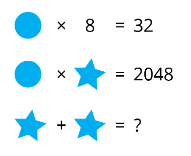Which of the following is the correct answer?

1. 64
2. 132
3. 512
4. 1024

Solution:

Looking at the first equation,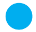\begin{align}​​\\[2ex] &= 32 \div 8\\[2ex] &= 4 \end{align}

Looking at the second equation

 $$4$$ $$\times$$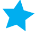$$=$$ $$2048$$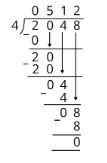$$=$$ $$2048 \div 4$$ $$=$$ $$512$$$$+$$$$=$$ $$512 + 512$$ $$=$$ $$1024$$

(4) 1024

Continue Learning
Multiplication Whole Numbers
Multiplication And Division Decimals
Model Drawing Strategy Division
Fractions Factors And Multiples
Area And Perimeter 1 Line Graphs
TimePrimaryPrimary 1Primary 2Primary 3Primary 4EnglishMaths
Multiplication
Whole Numbers
Line Graphs
Time
Multiplication And Division
Decimals
Model Drawing Strategy
Division
Fractions
Factors And Multiples
Area And Perimeter 1SciencePrimary 5Primary 6SecondarySecondary 1Secondary 2Secondary 3Secondary 4